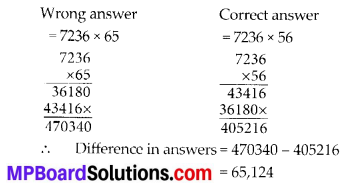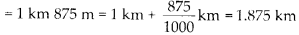## MP Board Class 6th Maths Solutions Chapter 1 Knowing Our Numbers Ex 1.2

Question 1.
A book exhibition was held for four days in a school. The number of tickets sold at the counter on the first, second, third and final day was respectively 1094, 1812, 2050 and 2751. Find the total number of tickets sold on all the four days.
Solution:
Number of tickets sold on first day = 1,094
Number of tickets sold on second day = 1,812
Number of tickets sold on third day = 2,050
Number of tickets sold on fourth day =2,751
Total tickets sold = 1,094 + 1,812 + 2,050 + 2,751 = 7,707
Therefore, 7,707 tickets were sold on all the four days.Question 2.
Shekhar is a famous cricket player. He has so far scored 6980 runs in test matches. He wishes to complete 10,000 runs. How many more runs does he need?
Solution:
Number of runs to achieve = 10,000
Number of runs scored = 6,980
Number of runs required = 10,000 – 6,980 = 3,020
Therefore, Shekhar needs 3,020 more runs.

Question 3.
In an election, the successful candidate registered 5,77,500 votes and his nearest rival secured 3,48,700 votes. By what margin did the successful candidate win the election?
Solution:
Number of votes secured by successful candidate = 5,77,500
Number of votes secured by his nearest rival = 3,48,700
Margin between them = 5,77,500 – 3,48,700 = 2,28,800
Therefore, the successful candidate won by a margin of 2,28,800 votes.Question 4.
Kirti bookstore sold books worth ₹ 2,85,891 in the first week of June and books worth ₹ 4,00,768 in the second week of the month. How much was the sale for the two weeks together? In which week was the sale greater and by how much?
Solution:
Worth of books sold in first week = ₹ 2,85,891
Worth of books sold in second week = ₹ 4,00,768
Total worth of books sold = ₹ (2,85,891 + 4,00,768)
= ₹ 6,86,659
Since, 4,00,768 > 2,85,891
Therefore, sale of second week is greater than that of first week by ₹ (4,00,768 – 2,85,891)
= 11,14,877

Question 5.
Find the difference between the greatest and the least number that can be written using the digits 6,2, 7,4, 3 each only once.
Solution:
Greatest five-digit number using digits 6,2,7,4,3 = 76432
Smallest five-digit number using digits 6,2,7,4,3 = 23467
Difference = 76432 – 23467 = 52965 Therefore, the difference is 52,965.

Question 6.
A machine, on an average, manufactures 2,825 screws a day. How many screws did it produce in the month of January 2006?
Solution:
Number of screws manufactured in one day = 2,825
Number of screws manufactured in the month of January (31 days) = 2,825 × 31 = 87,575
Therefore, the machine produced 87,575 screws in the month of January.Question 7.
A merchant had ₹ 78,592 with her. She placed an order for purchasing 40 radio sets at ? 1200 each. How much money will remain with her after the purchase?
Solution:
Cost of one radio set = ₹ 1200 Cost of 40 radio sets = ₹ (1200 × 40)
= ₹ 48,000
Now, total money with merchant = ₹ 78,592
Money left with her = ₹ (78,592 – 48,000)
= ₹ 30,592
Therefore, ₹ 30,592 will remain with her after the purchase.

Question 8.
A student multiplied 7236 by 65 instead of multiplying by 56. By how much was his answer greater than the correct answer? (Hint: Do you need to do both the multiplications?)
Solution:Question 9.
To stitch a shirt, 2 m 15 cm cloth is needed. Out of 40 m cloth, how many shirts can be stitched and how much cloth will remain?
(Hint: convert data in cm.)
Solution:
Cloth required to stitch one shirt = 2 m 15 cm = 2 × 100 cm + 15 cm = 215 cm
Length of cloth = 40 m = 40 × 100 cm = 4000 cm
Number of shirts can be stitched – 4000 ÷ 215Therefore, 18 shirts can be stitched and 130 cm (1 m 30 cm) cloth will remain.

Question 10.
Medicine is packed in boxes, each weighing 4 kg 500g. How many such boxes can be loaded in a van which cannot carry beyond 800 kg?
Solution:
The weight of one box = 4 kg 500 g = 4 × 1000 g + 500 g = 4500 g
Maximum load can be loaded in a van = 800 kg = 800 × 1000 g = 800000 g
Number of boxes = 800000 ÷ 4500Therefore, 177 boxes can be loaded in the van.

Question 11.
The distance between the school and the house of a student is 1 km 875 m.
Everyday she walks both ways. Find the total distance covered by her in six days.
Solution:
Distance between the school and houseTotal distance covered in one day
= (1.875 × 2)km = 3.750 km
Distance covered in six days = (3.750 × 6) km
= 22.500 km
Therefore, a student covered 22 km 500 m distance in six days.Question 12.
A vessel has 4 litres and 500 ml of curd. In how many glasses, each of 25 ml capacity, can it be filled?
Solution:
Quantity of curd in a vessel = 4 litres 500 ml = 4 × 1000 ml + 500 ml = 4500 ml
Capacity of one glass = 25 ml
Number of glasses can be filled = 4500 ÷ 25Therefore, 180 glasses can be filled by curd.